# I can't discretize a PIPD controller

#### vra

Hi all, how are you?.

I'm designing a PIPD controller for a BLDC motor, I have almost everything designed but I'm having problems with the code of the function that calculates the duty cycle of my PWM.

The problem is that I've made a bad code of the function that calculates the PIPD control action, then I went to the writing desk again and checked my function to see what happened and I've noticed that the algorithm was bad.

then I remade the controller which can be seen in the image bellow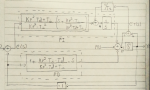what your seeing in the sketch above are the PI and PD control actions with an anti windup feedback, that without the andto windup feedback works perfectly in continous mode, the anti windup feedback is for the discrete system and preciselly there is the problem. Like the anti windup feedback must pass through an integrator I spread the PI action with has an integrator included to avoid include another integrator in the system and make the anti windup feedback, is this valid?.

the other problem that I have is that I couldn't find a z-transform of the part of the PI action that has a zero and neither for the PD action to allow me make the equations in differences to make the controller algorithm.

Can anyone help me with those problems?.

NOTE: "G" stands for the plant transfer function.

Thanks in advance for the help.

#### pnachtwey

That doesn't look right. It looks too complicated for what you are trying to do.
I don't see why there is a need for Kp^2
Did you try using Tustin's approximation for converting from the s to the z domain?
This is easy.
Using matched z transforms is a little harder.
The PD block only seems to act on changes in the action position or velocity or what ever Y is.

•vra

#### vra

Y is velocity and actually PD intended to act in the velocity only, that is for definition of the method to avoid mess with the integral part of the control.

The Kp^2 comes fror the algebraic development of the method, the autor defines the constants of the method in a different form and I needed to develop it for what I want.

I will look for the Tustin aproximation that you suggested.

#### pnachtwey

Post a link to the derivation. It doesn't look right.
When in velocity mode, there is a need for an integrator. A PD controller will not reach the desired speed.
I think you need to study more from reliable sources.
A PI controller should be as simple as
Code:
``u(n)=u(n-1)+K0*E+K1*E(n-1)``
Where:
Code:
``````u(n) is the current control output
K0 = Ki* ΔT+Kp
K1=-Kp
E(n)  is the current error.``````
If the output is to be limited to +/- 100% then
Code:
``u(n)=max(min(u(n-1)+K0*E+K1*E(n-1),100),-100)``
This also avoids integrator windup and is very simple and fast but will suffer if the feed back has poor resolution.
A DSP will take only a few clock cycles do execute this.
I should make a video on this for my Peter Ponders PID YouTube channel..

#### vra

Post a link to the derivation. It doesn't look right.
When in velocity mode, there is a need for an integrator. A PD controller will not reach the desired speed.
I think you need to study more from reliable sources.
A PI controller should be as simple as
Code:
``u(n)=u(n-1)+K0*E+K1*E(n-1)``
Where:
Code:
``````u(n) is the current control output
K0 = Ki* ΔT+Kp
K1=-Kp
E(n)  is the current error.``````
If the output is to be limited to +/- 100% then
Code:
``u(n)=max(min(u(n-1)+K0*E+K1*E(n-1),100),-100)``
This also avoids integrator windup and is very simple and fast but will suffer if the feed back has poor resolution.
A DSP will take only a few clock cycles do execute this.
I should make a video on this for my Peter Ponders PID YouTube channel..
Well, the image that you see is the derivation made by my own hand and all the combination of factors Kp, Td and Ti resulted from the derivation, now, the definition that I'm using is the definition that comes from the Astrom book called "Control System Design", page 224, 2002 edition; and that (without the anti-windup feedback) works pefectly in continous simulation made with scilab. Bellow is an image with the controller definition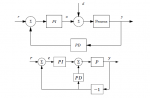the only difference between the block diagram above with my diagram is that I added the anti-windup feedback and previously separated the PI controller to input the anti-windup feeback before the integrator part of the PI controller, separation wich for what I'm not sure if is valid for this controller.

I'm sure that the scilab simulation is my own derivation because scilab doesn't have function to calculate Laplace transform so I needed to code every function with my own hands. Attached bellow is an image with the result if the simulation where I coded myself several controllers and the PIPD controller that I show in the initial image works smoothly (without anti-windup feedback in the scilab simulation), without overshoot and with the minimun stable error possible (see the blue curve that reaches the set point very quickly).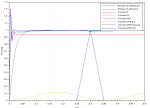about the link to the derivation I don't have it, I have it in paper also made by my own hand and from that I coded it in scilab, I only have the problem that initially I coded the controller very different to what I have because I made mistakes discretizing the system with the controller included in that time.

#### pnachtwey

OK, I can see what you are trying to do now if you follow the book. What you are doing is making a special kind of PID controller with two proportional gains. One that acts on the error and is in the forward path that can place the zero and another in the feed back path that places the closed loop pole. The derivative gain only acts on changes in the feed back path.
Your original diagram doesn't make sense compared to the one in the Astrom book. On top of that I still don't see how you get terms like Kp^2.

The last graph looks a little strange. The part that looks like triangle doesn't look right. The Control I-PD doesn't look like it is tuned at all.

DONT USE Z-N for motion control! Use pole placement! Also in your case you need to learn how to place the zeros too because you have two proportional gains. Zeros are good for extending bandwidth but they should be placed near and to the left of any closed loop poles in the s-plane.

There shouldn't be the extreme overshoot of the reference speed.
Motion controllers rarely use time constants to express gains. Motion controllers usually use true gains so that the derivative gain is multiplied by the error between the target and actual velocity since you are controlling velocity.

I can do this for you but that wouldn't be any fun. I would like to see how you get terms like Kp^2
It shouldn't be that difficult.

I/we make motion controllers.

You really should look at my YouTube channel Peter Ponders PID. I cover system identification and pole placement.
I have plenty of example with all the math shown.

Scilab is OK but I prefer Python. Scilab is a kludge.

#### vra

The signal that looks like a triangle is a disturbance, thats why it doesn't look good and yes, the I-PD isn't tunned at all, what I made there was to apply the same calculated constants to all methods to see what method works better without tunning them, from there I chose the PIPD controller because its the one that gave the better result of all used methods.

The derivation of the terms to obtain Kp^2 comes from the definition (also from the book) shown in the following image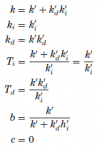and the following image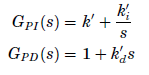after that I simply made the transfer functions of both paths what gaves me the following

Y(S)/R(S) = [PI(S) * G(S)] / [1 + (PD(S) * PI (S) * G(S)])

where

Y(S): output of the system.
R(S): Input of the system.
PI(S): proportional-integral action.
PD(S): Proportional-derivative action.
G(S): original system without controller.

after that I simply substituded KP, Ti and Td (previously calculated) in the first image shown in this reply and the result was replaced in the relations of the second image of this replay, that made me transfer functions for PD and PI shown in the first image of the thread.

and yes, I must have some error around there because I had a lot of years without making nothing of control systems (not an excuse) until I started with this project about six months ago (actually the learning design of the PID controller was started about two months ago), now I can start, stop and change the rotor direction of the motor by code but the most important part what is the controller part (the ten lines of code that makes the magic) doesn't work and the motor starts to fail.

#### pnachtwey

That still doesn't look right. There must be a miss print. There should be no Kp^2
This looks OK
Y(S)/R(S) = [PI(S) * G(S)] / [1 + (PD(S) * PI (S) * G(S)])
But
why not just Y(s)/R(s)=(Ki/s+Kp)*G(s)/(1+(Ki/s+Kp+Kd*s)*G(s))?
basically the derivative gain is only acting on changes in the actual velocity whereas the Ki and Kp are acting on the error between the target and actual velocity.

Another point. Why use a derivative gain? A derivative gain would only be necessary if the system has two open loop poles. Second. Your form of PIPD will not work well if the poles are complex. ( the system is under damped ). A hydraulic motor controlling a heavy load would fit in this category.

You only need a PI controller for velocity control if the motor and load act like they have only one pole ( time constant ).
Basically, you need to have one gain, beside the integrator gain, for each open loop pole. The integrator has its own pole so it doesn't count.

Here is an example controlling a simple one pole system in position mode.
Integrating the velocity to position adds another pole so there are 2 poles so a proportional and derivative gain is required. The integrator comes with its own pole so altogether the closed loop systems has 3 poles.
Notice that I can compute formulas for the gains that should result in a critically damped response. It doesn't because there are zeros closer to the origin than the poles.

Unlike the people that write books, I have spent many years actually doing motion control. I really doubt the Kp^2 in the Astrom book is right. You don't see any Kp^2 in my example and it works.

#### vra

That still doesn't look right. There must be a miss print. There should be no Kp^2
This looks OK
Y(S)/R(S) = [PI(S) * G(S)] / [1 + (PD(S) * PI (S) * G(S)])
But
why not just Y(s)/R(s)=(Ki/s+Kp)*G(s)/(1+(Ki/s+Kp+Kd*s)*G(s))?
basically the derivative gain is only acting on changes in the actual velocity whereas the Ki and Kp are acting on the error between the target and actual velocity.

Another point. Why use a derivative gain? A derivative gain would only be necessary if the system has two open loop poles. Second. Your form of PIPD will not work well if the poles are complex. ( the system is under damped ). A hydraulic motor controlling a heavy load would fit in this category.

You only need a PI controller for velocity control if the motor and load act like they have only one pole ( time constant ).
Basically, you need to have one gain, beside the integrator gain, for each open loop pole. The integrator has its own pole so it doesn't count.

Here is an example controlling a simple one pole system in position mode.
Integrating the velocity to position adds another pole so there are 2 poles so a proportional and derivative gain is required. The integrator comes with its own pole so altogether the closed loop systems has 3 poles.
Notice that I can compute formulas for the gains that should result in a critically damped response. It doesn't because there are zeros closer to the origin than the poles.

Unlike the people that write books, I have spent many years actually doing motion control. I really doubt the Kp^2 in the Astrom book is right. You don't see any Kp^2 in my example and it works.
Ok, I will analize and try what you said and I´ll be communication any advance.

#### vra

I forgot to ask, is there any python api or program to work with control systems?

#### vra

Hey, I realized all the warnings you gave me with the original system that I published, the terrible mistake that I had with the implementation of the anti-windup feedback, about the constants I don't see any mistake but I'll check one more time.

#### pnachtwey

I forgot to ask, is there any python api or program to work with control systems?
Yes, it is basically a copy of the Matlab library with a few adjustments due to the differences in the languages.